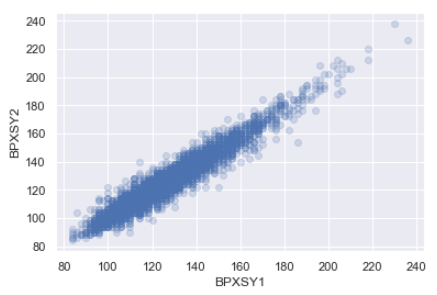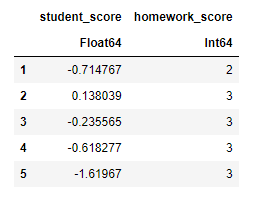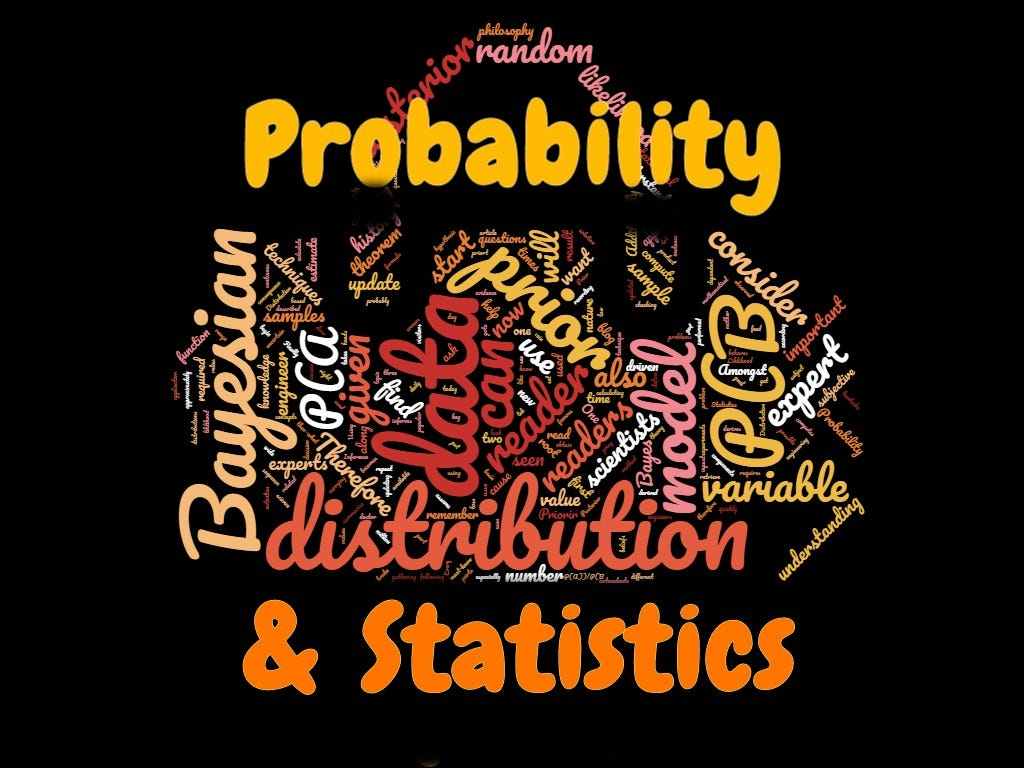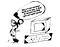1595096220

# Factors That Can Contribute to the Faulty Statistical Inference

Hypothesis testing is a procedure where researchers make a precise statement based on their findings or data. Then, they collect evidence to falsify that precise statement or claim. This precise statement or claim is called the null hypothesis. If the evidence is strong to falsify the null hypothesis, we can reject the null hypothesis and adapt the alternative hypothesis. This is the basic idea of hypothesis testing.

## Error Types in Statistical Testing

There are two distinct types of errors that can occur in formal hypothesis testing. They are:

Type I: Type I error occurs when the null hypothesis is true but the hypothesis testing results show the evidence to reject it. This is called a false positive.

Type II: Type II error occurs when the null hypothesis is not true but it is not rejected in hypothesis testing.

Most hypothesis testing procedure performs well controlling type I error (at 5%) in ideal conditions. That may give a false idea that there is only a 5% probability that the reported findings are wrong. But it’s not that simple. The probability can be much higher than 5%.

## Normality of the Data

The normality of the data is an issue that can break down a statistical test. If the dataset is small, the normality of the data is very important for some statistical processes such as confidence interval or p-test. But if the data is large enough, normality does not have a significant impact.

## Correlation

If the variables in the dataset are correlated with each other, that may result in poor statistical inference. Look at this picture below:In this graph, two variables seem to have a strong correlation. Or, if a series of data is observed as a sequence, that means values are correlated with its neighbors, and there may have some clustering or autocorrelation in the data. This kind of behavior in the dataset can adversely impact the statistical tests.

## Correlation and Causation

This is especially important when interpreting the result of a statistical test. “Correlation does not mean causation”. Here is an example. Suppose, you have study data that shows, more people who do not have college education believe that women should get paid less than men in the workplace. You may have conducted a good hypothesis testing and prove that. But care must be taken on what conclusion is drawn from this. Probably, there is a correlation between college education and the belief that ‘women should get paid less’. But it is not fair to say that not having a college degree is the cause of such belief. This is a correlation but not a direct cause ad effect relationship.

A more clear example can be provided from medical data. Studies showed that people with fewer cavities are less likely to get heart disease. You may have enough data to statistically prove that but you actually cannot say that the dental cavity causes heart disease. There is no medical theory like that.

#statistical-analysis #statistics #statistical-inference #math #data analysis

## Buddha Community1595096220

## Factors That Can Contribute to the Faulty Statistical Inference

Hypothesis testing is a procedure where researchers make a precise statement based on their findings or data. Then, they collect evidence to falsify that precise statement or claim. This precise statement or claim is called the null hypothesis. If the evidence is strong to falsify the null hypothesis, we can reject the null hypothesis and adapt the alternative hypothesis. This is the basic idea of hypothesis testing.

## Error Types in Statistical Testing

There are two distinct types of errors that can occur in formal hypothesis testing. They are:

Type I: Type I error occurs when the null hypothesis is true but the hypothesis testing results show the evidence to reject it. This is called a false positive.

Type II: Type II error occurs when the null hypothesis is not true but it is not rejected in hypothesis testing.

Most hypothesis testing procedure performs well controlling type I error (at 5%) in ideal conditions. That may give a false idea that there is only a 5% probability that the reported findings are wrong. But it’s not that simple. The probability can be much higher than 5%.

## Normality of the Data

The normality of the data is an issue that can break down a statistical test. If the dataset is small, the normality of the data is very important for some statistical processes such as confidence interval or p-test. But if the data is large enough, normality does not have a significant impact.

## Correlation

If the variables in the dataset are correlated with each other, that may result in poor statistical inference. Look at this picture below:In this graph, two variables seem to have a strong correlation. Or, if a series of data is observed as a sequence, that means values are correlated with its neighbors, and there may have some clustering or autocorrelation in the data. This kind of behavior in the dataset can adversely impact the statistical tests.

## Correlation and Causation

This is especially important when interpreting the result of a statistical test. “Correlation does not mean causation”. Here is an example. Suppose, you have study data that shows, more people who do not have college education believe that women should get paid less than men in the workplace. You may have conducted a good hypothesis testing and prove that. But care must be taken on what conclusion is drawn from this. Probably, there is a correlation between college education and the belief that ‘women should get paid less’. But it is not fair to say that not having a college degree is the cause of such belief. This is a correlation but not a direct cause ad effect relationship.

A more clear example can be provided from medical data. Studies showed that people with fewer cavities are less likely to get heart disease. You may have enough data to statistically prove that but you actually cannot say that the dental cavity causes heart disease. There is no medical theory like that.

#statistical-analysis #statistics #statistical-inference #math #data analysis1603098000

## Dataset

Suppose we want to study the effect of student diligence and homework quality. Let’s imagine that we were somehow able to assign a real number to measure a student’s diligence and that homework quality is measured on a 10-point scale, 0 to 10.In other words, our dataset looks like this:Figure 1: Sample synthetic dataset

A student’s student score is just a random variable sampled from a standard normal distribution.

#statistical-inference #data-science #mathematics #statistics #bayesian-statistics1603018800

## Introduction

Let’s divide the universe of models into two types: statistical and scientific. Both classes of models aims to understand the relationship between a target variable i.e. the y, and a set of features i.e. the **x. **The former aims to find statistically sound relationships based on data while the latter has the property of being able to describe a cause and effect relationship.

All the examples in this series have used statistical models e.g. GLMs to illustrate a concept in Bayesian inference. However, Bayesian inference is just as relevant to building scientific models.

This article will show how to incorporate Bayesian inference to build scientific models and the benefits of doing so.

The code to reproduce the results described in this article can be found in this notebook.

## Problem

To keep the math simple, let’s imagine we want to build a model to predict a person’s weight given height.

#statistics #machine-learning #data-science #bayesian-statistics #bayesian-inference1596919440

## Understanding Statistics And Probability: Bayesian Inference

Bayesian inference is one of the most popular statistical techniques. It is a technique whereby the prior probabilities of an event are updated when the new data is gathered. Bayesian inference is a data-driven technique.

The Bayesian models are traditionally one of the first models to use. They are used as the baseline models as they are based on the simplistic view of the world and enable the scientists to explain the reasoning easier. Consequently, Bayesian inference is one of the most important techniques to learn in statistics.An important concept of Probability And Statistics

## Article Aim

1. What Is Bayesian Inference?
2. What Is Baye’s Theorem?
3. Examples To Understand The Concepts
4. Naive Bayes Model

Bayesian inference is used in a large number of sectors including insurance, healthcare, e-commerce, sports, law amongst others. Bayesian inference is heavily used in classification algorithms whereby we attempt to classify/group text or numbers into their appropriate classes.

Furthermore, it is growing in interest in banking and in particular in the finance sector.

## 1. What is Bayesian Inference?

Before I explain what Bayesian Inference is, let’s understand the key building blocks first.

I will start by illustrating an example.

### Computer Engineers Example

Let’s consider that I have a computer that stopped working. There are two computer engineers in my neighborhood who can fix the computer.

Both of the engineers claim to have different techniques to diagnose and fix the problem.

### First Engineer — Frequentist Approach

The first engineer has a model, made up of a mathematical equation. This model is built based on the frequency of an event. The model requires a set of inputs to compute the diagnosis of why the computer stopped working.

The way the first engineer diagnoses the problem is by asking questions that the model requires as inputs.

As an instance, the engineer would ask about the computer specification such as the operating system, hard disk size and processor name. He would then feed the answers to the model and the model would then give the reasons of why the computer broke down.

The model will use the observed frequency of the events to diagnose why the computer stopped working.#statistics #bayes-theorem #probability #data-science #bayesian-inference #data analysis1624679160

## Introduction To Infer.NET - A Framework For Probabilistic Programming in DOTNET

### Introduction

Infer.NET is a framework for making Bayesian inference on graphical models. The user specifies the factors and variables of a graphical model. Infer.NET analyses them and creates a schedule for making inference on the model. The model can then be queried for marginal distributions.

Microsoft Research and .NET Foundation developed Infer.NET. It is also a part of ML.NET machine learning framework. (Read this article if you are unfamiliar with ML.NET).

Originally written in C# language, the development of Infer.NET began Cambridge, UK in 2004. It was initially released for academic purposes in 2008 and then was open-sourced in 2018.

Infer.NET is used internally at Microsoft as the machine learning engine in some of its products such as OfficeAzure, and Xbox. It is already in use in several projects related to social networking, healthcare, computational biology, web search, machine vision, etc. It enables the creation and handling of complex models in these projects with just a few lines of code.

Before moving on to the details of Infer.NET, let us first understand some of the underlying concepts.

#developers corner #.net framework #bayesian inference #infer.net #probabilistic programming #introduction to infer.net